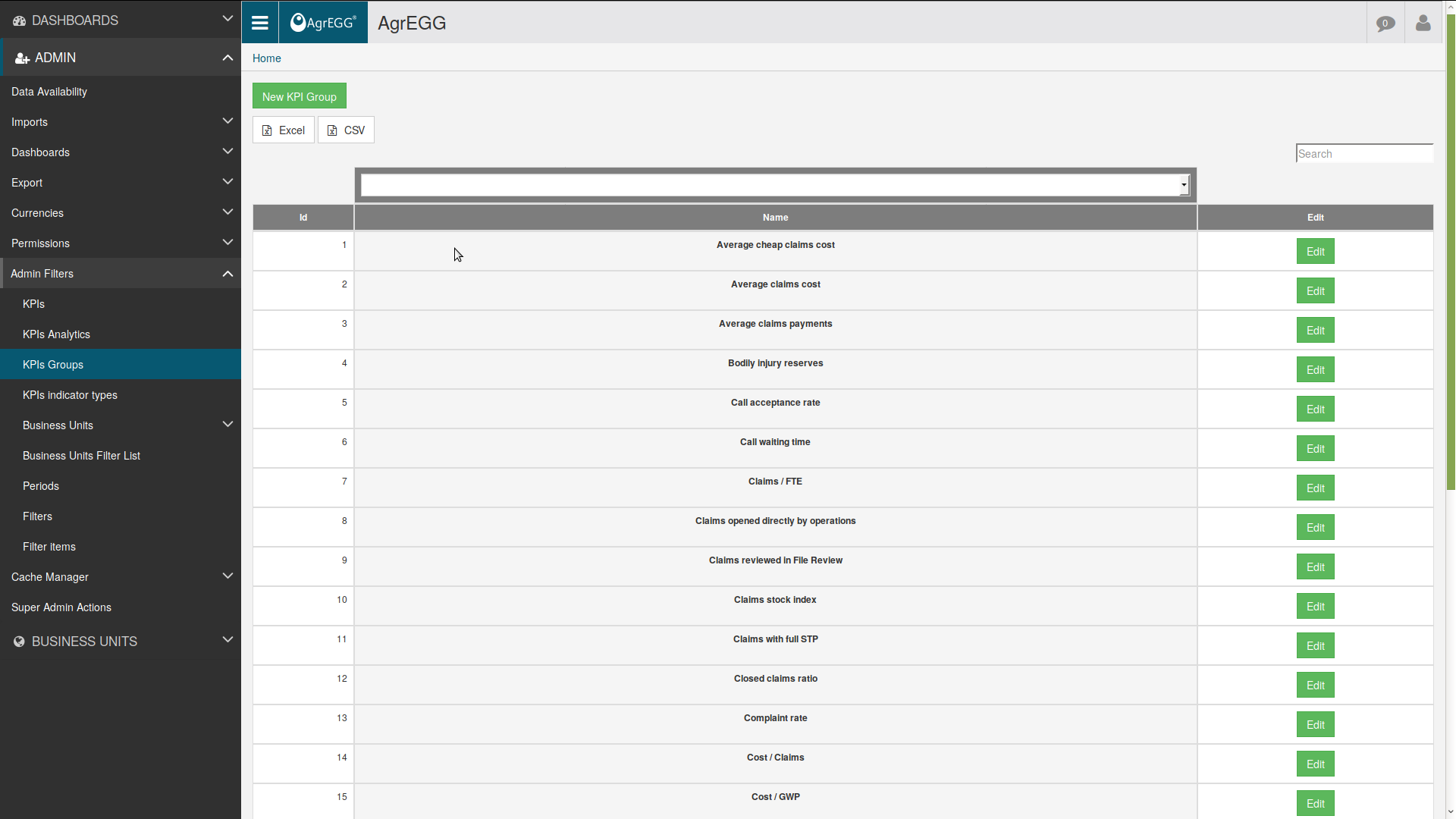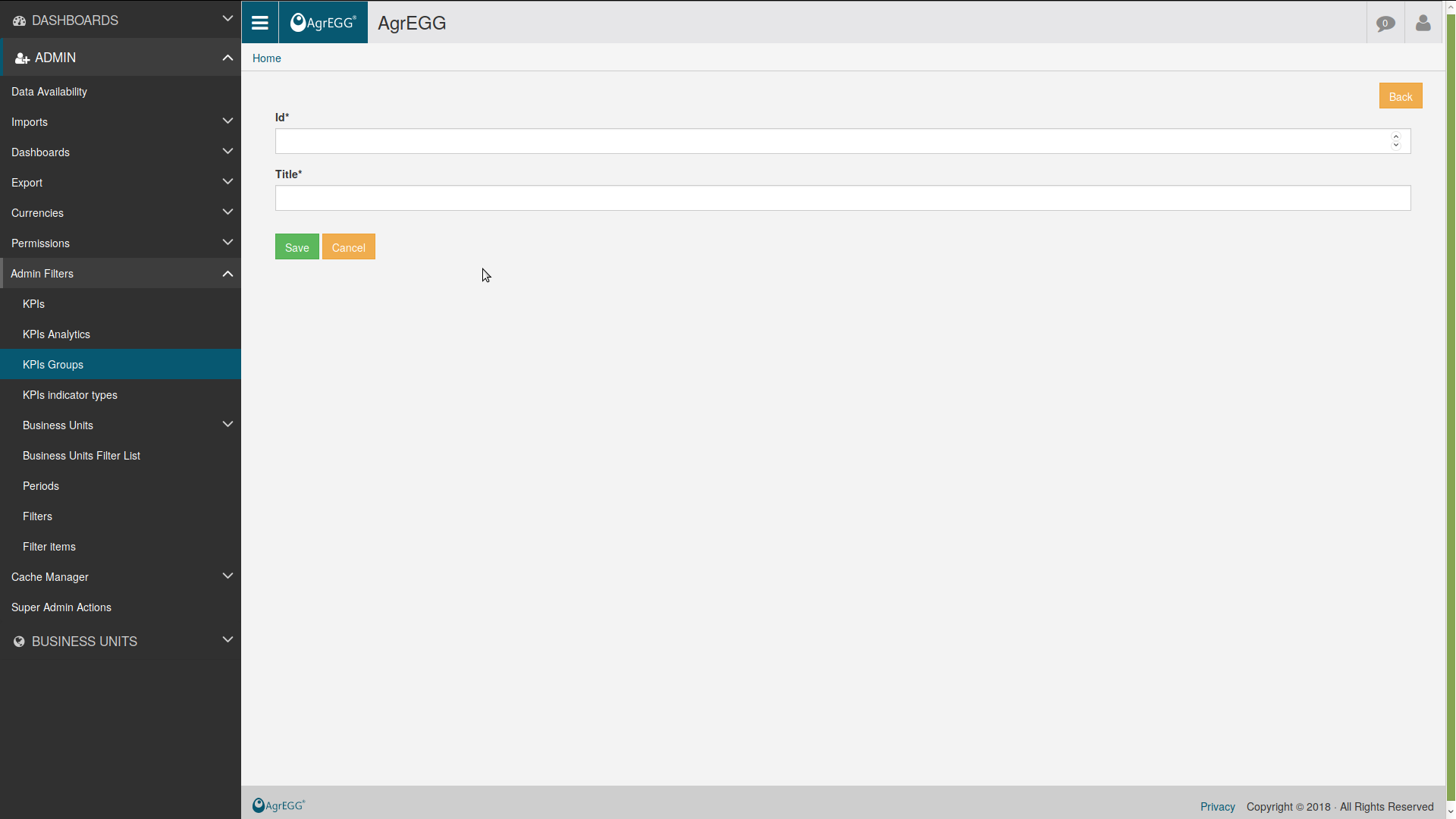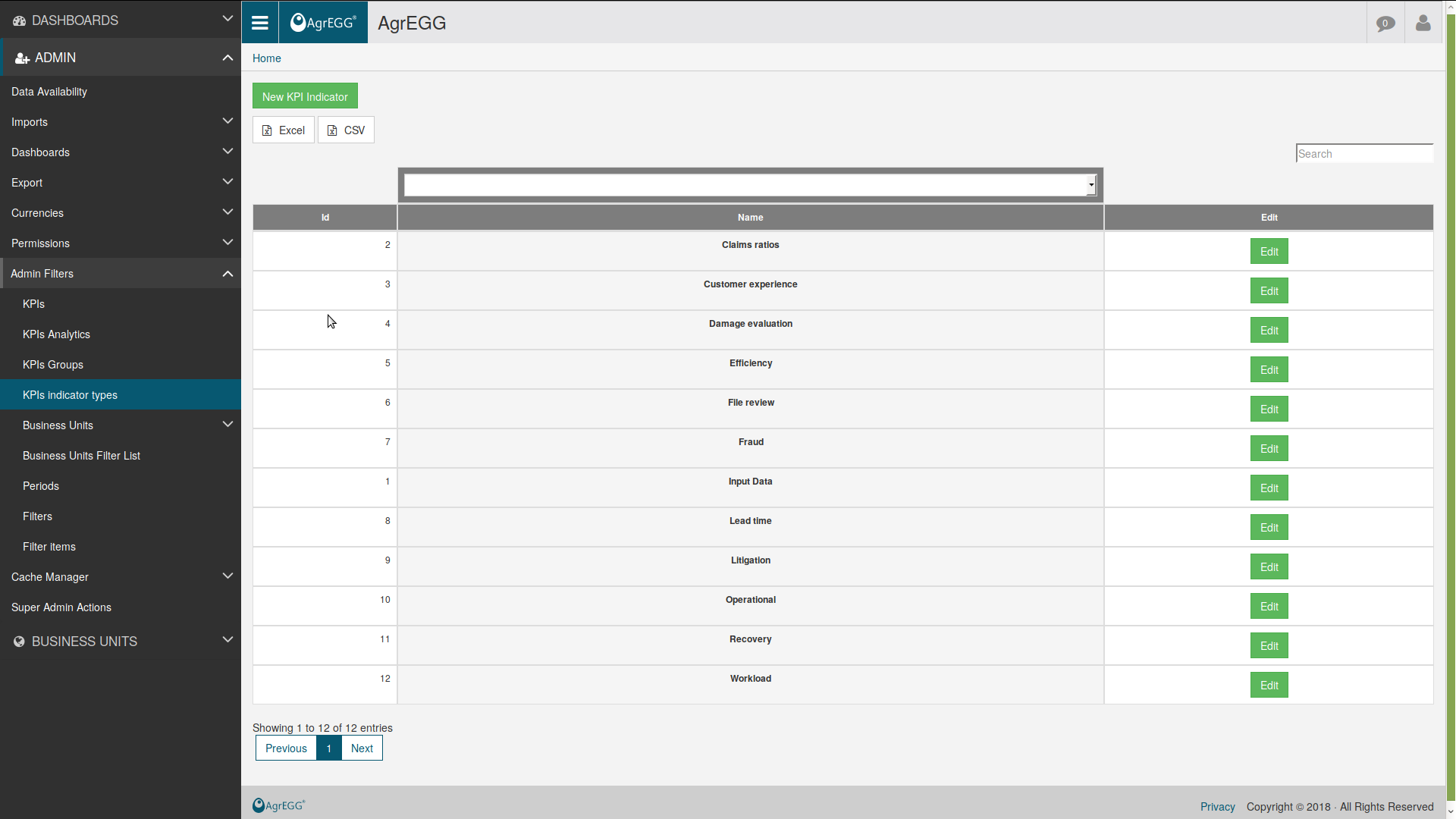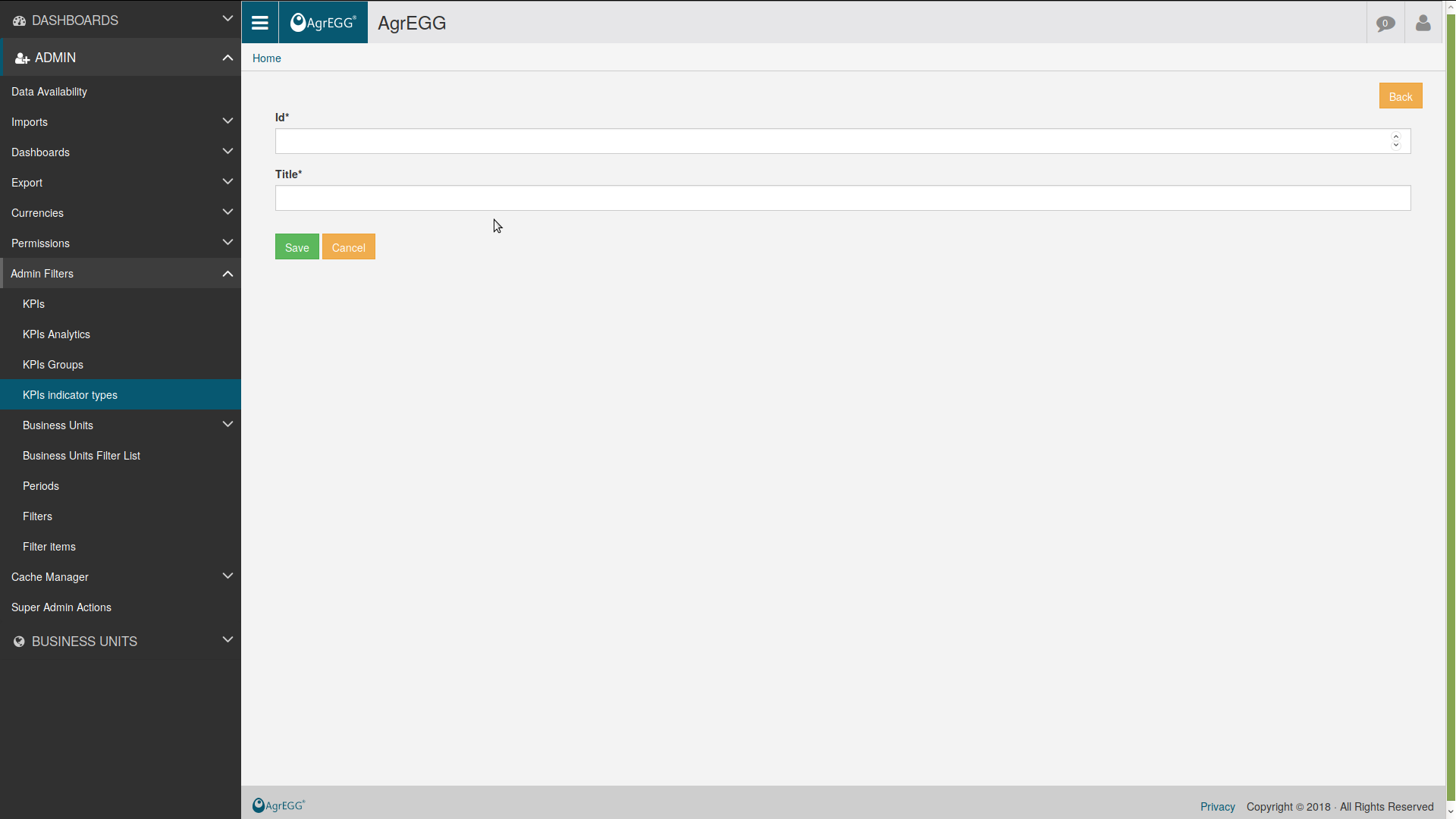# KPIs¶

KPIs are the central numerical quantities which are analyzed in a dashboard.

## Create a KPI¶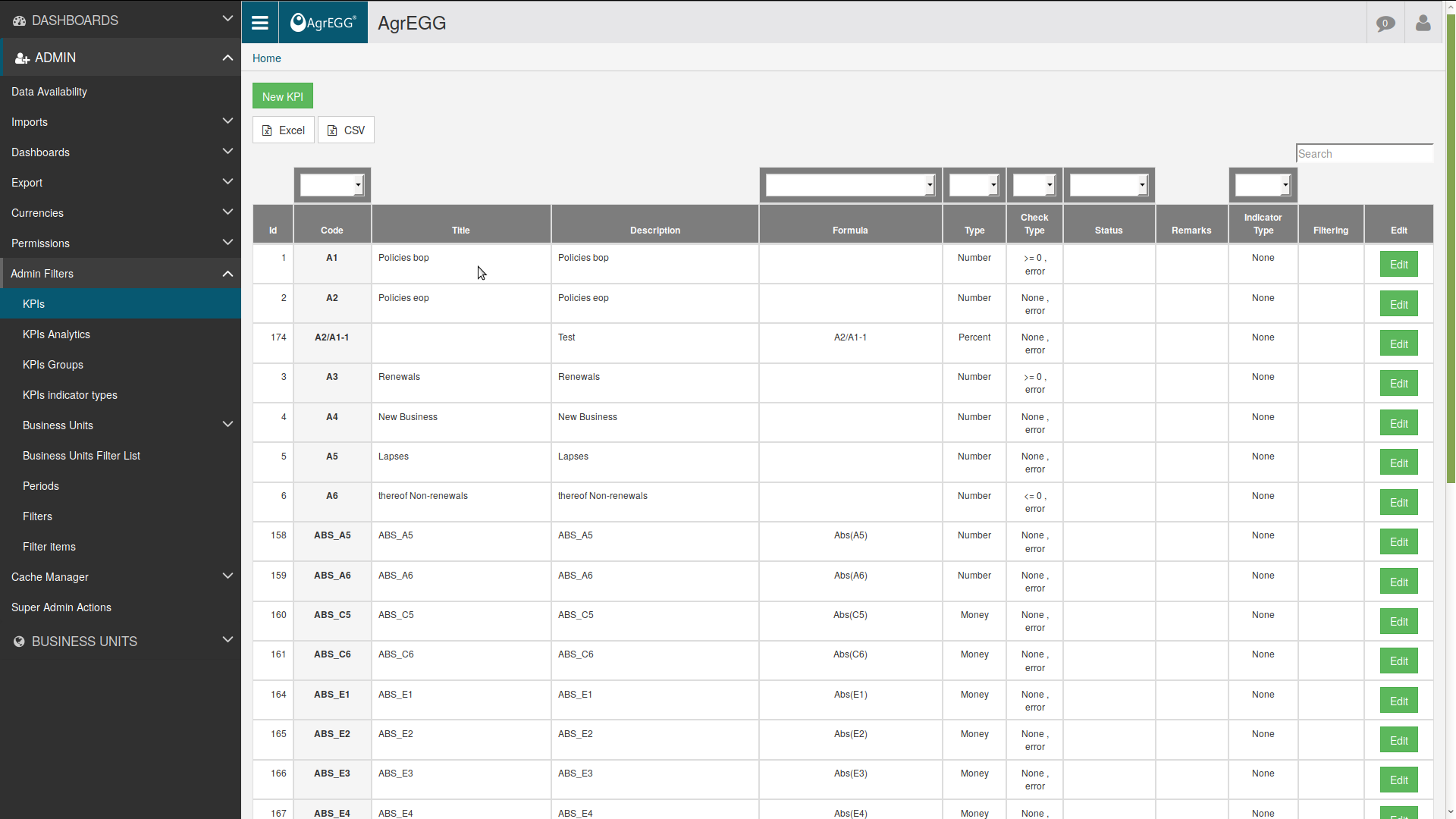Click “New KPI” to create a new KPI, or “Edit” to edit an existing one, with the following fields:

• Description: simple human-readable description of the KPI
• Code: univocal string (max 10 characters)
• Import type: select from the drop-down menu the corresponding import type
• Group: select from the drop-down menu (see below)
• Indicator type: select from the drop-down menu (see below)
• Measure type: select from the drop-down menu if integer, decimal, money, or percent
• Aggregation type: select from the drop-down menu how the KPI is aggregated when built from many numbers
• Check Type: select from the drop-down menu what kind of check should be performed on its value
• Value Scale: set a scale (useful for very small or large quantities)
• Value Scale Output: similar as above
• Period type: select from the drop-down menu if actual, forecast, or target
• Projection (years): if period type is not actual, set how many years in the future the KPI is shown
• Color: when drill-down level is zero, use this color
• Number of decimals: number of decimal digits to show for a decimal number
• Reset the column and start from zero: in advanced walk plot, set if kpi starts from level 0 or is the difference with the previous KPI
• Formula: can be used to create new KPIs starting from existing ones, by use of mathematical formulas
• Json Parameter: additional parameters can be set here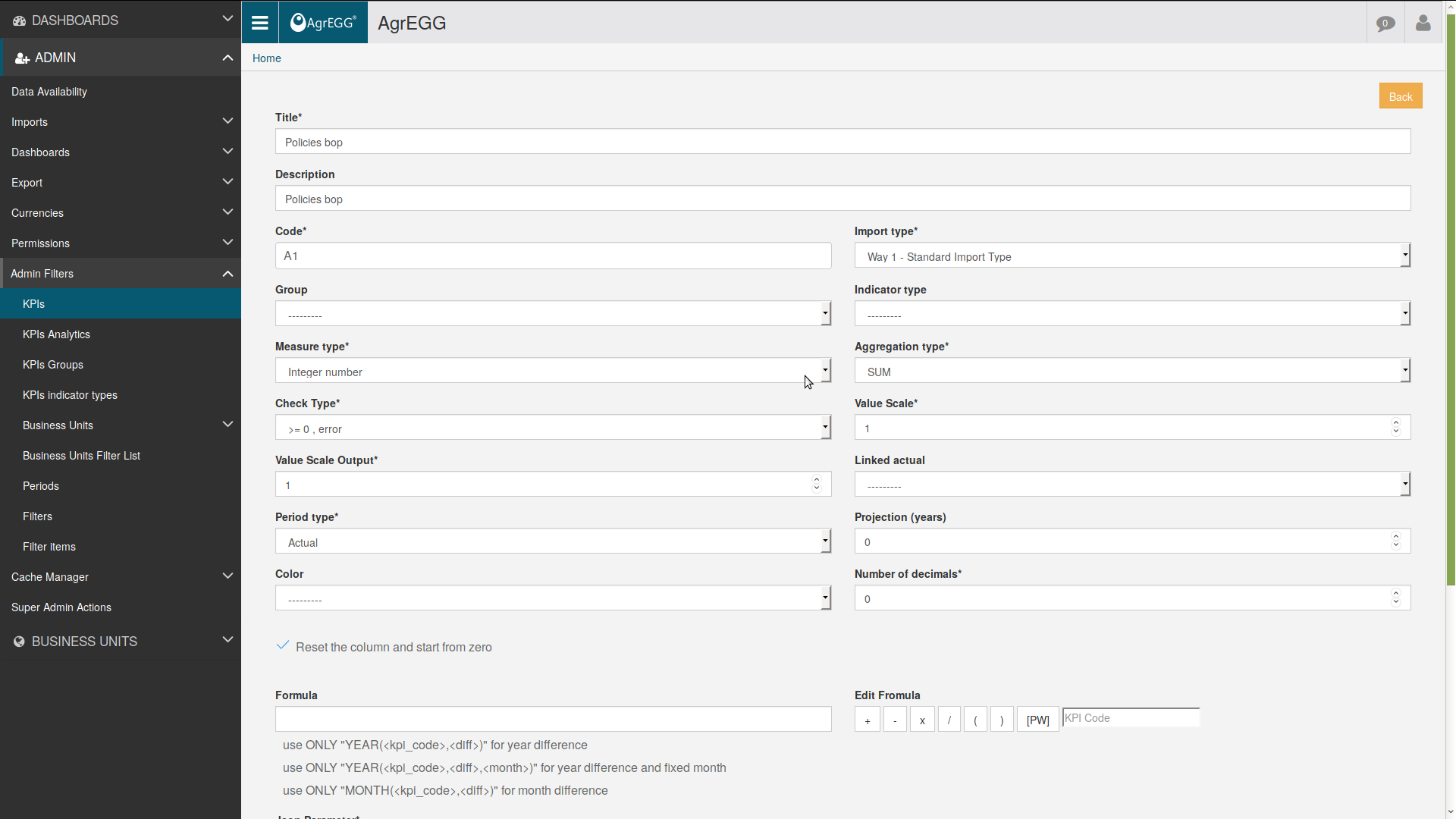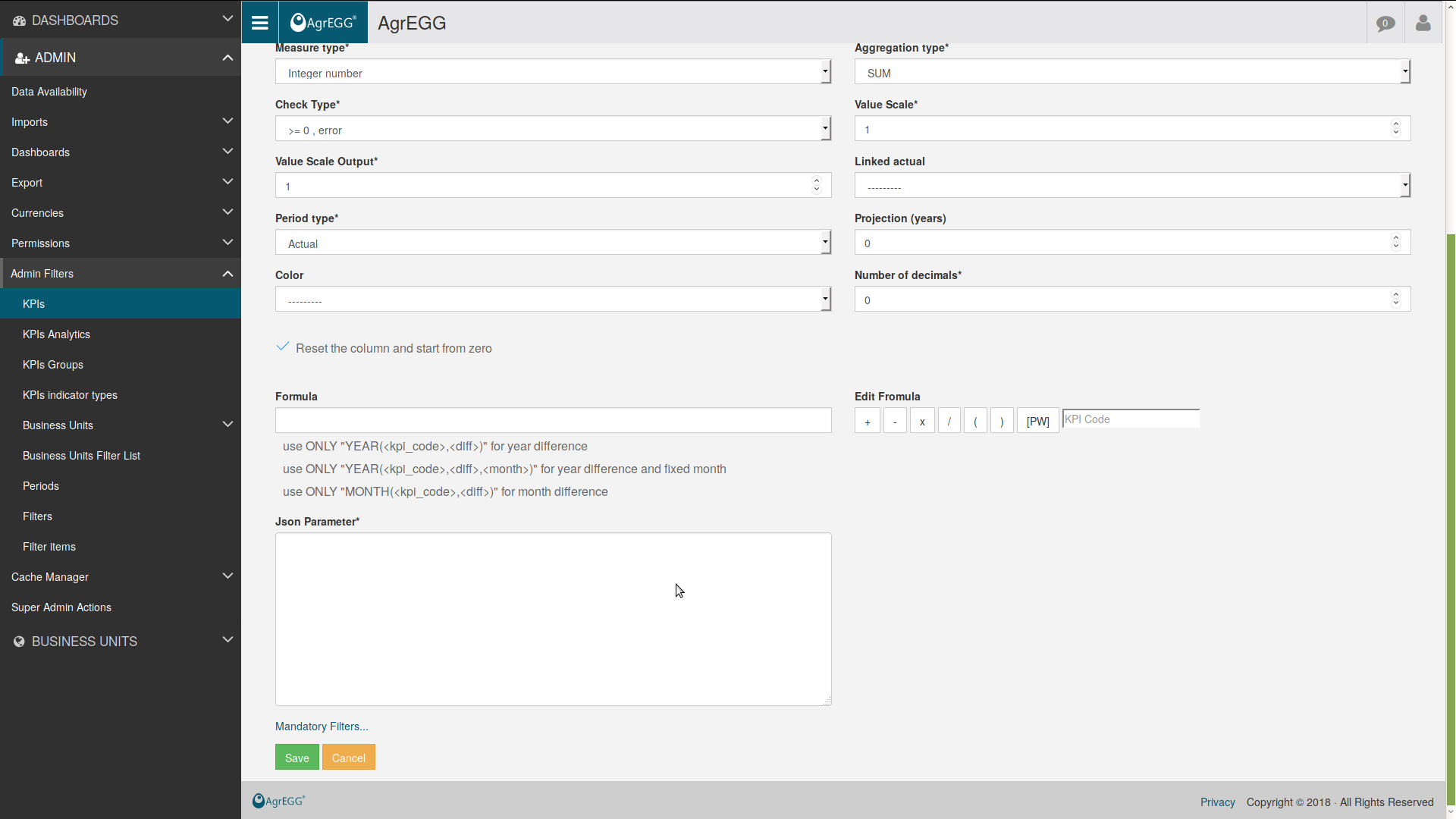In Json Parameter set the following values to force the calculation in a given way.

```{
"gpy":"2",
"period": "all",
"get_all_months_period": True
}
```

This sums its value for a single year:

```"gpy":"2",
"period": "year_2017"
```

This sums its value for the previous year and for a specific month “pyearm_X_Y” (“get_all_months_period” sets if all months of the given period are considered): X is the number of previous years and Y is the id of the period type

```"gpy":"2",
"period": "pyearm_1_12",
"get_all_months_period" : True
```

This sums its value for the previous year “pyear_X” (“get_all_months_period” sets if all months of the given period are considered):

```"gpy": "2",
"period": "pyear_2",
"get_all_months_period" : "1"
```

This get X year for the same period type (e.g. december) of the latest selected period use before “nyear_X”. With this selection, if you select August 2018, the system will return also August 2017, 2016, …

```"period": "nyear_2",
```

To take all periods before the last selected one, set “period” = “all_from_last”. To take all periods before the laset selected one in the given year, set “period” = “all_current_year_from_last”. To take all periods before the laset selected one in the previous year, set “period” = “all_previous_year_from_last”. To cumulate, set “cumulate” = “true”.

Let’s switch to plots.

This sums a kpi value for all periods specified in “period”:

```"gpy": False,
"period": "260"
```

## KPI Groups¶